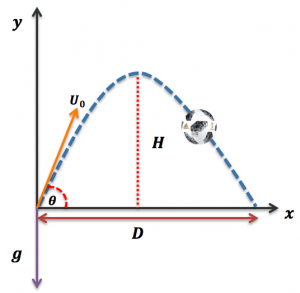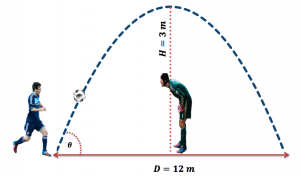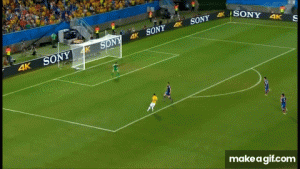# The maths behind a chip goal

We analyse the maths and physics required to execute a good chip shotSwitzerland vs. Argentina, 29th February 2012, Wikimedia Commons, CC BY 3.0

One week has passed since the World Cup began, and it has been full of exciting and unexpected moments. We are so excited by all the action that we decided to bring you another football blog (check out our previous one here). This time we will be talking about a popular and beautiful shot called the chip shot, commonly executed by world class players such as Lionel Messi.

I have always said that the most talented players are literally football machines, as they know the perfect conditions necessary to kick the ball with extremely high precision and the desired effect to score amazing goals. It seems that they do a quick computation in their heads, considering the environmental conditions to decide how strong or light the ball must be hit. The most remarkable example of this is the chipped goal, one of many difficult shots to pull off in football.Imagine you are in the field, your team is losing but you are organising a counter attack. You sprint and put yourself in an excellent attacking position. After dribbling some opponents, your teammate makes an outstanding cross that finds you. It is only you and the goalkeeper, who is quickly approaching you to restrict your shooting angle. You notice that you can make a chip shot so the ball will go over the keeper’s head and will land in the back of the net. There are two scenarios: you calculate your shot perfectly and it will make the ball travel with a parabolic trajectory that is impossible for the keeper to catch. You will be the hero and your goal will be on the news. But one wrong calculation will make you fail and waste a valuable opportunity. Sounds difficult, doesn’t it?

As we have stated in our previous blogs, and according to Newton’s 2nd law, the trajectory of a ball can be affected by three main forces: the gravitational force $F_G$, the lift force (associated with a spinning object), $F_L$ and the drag force (or air resistance), $F_D$. In order to observe this fantastic and eye-catching curve, the following conditions need to be satisfied: the first one is that the ball has to be hit with an initial velocity smaller than $20$ m/s. If the player kicks the ball with a greater velocity, the chip effect might not be achieved. This condition is crucial to satisfy the remaining conditions, which are that the gravitational force $F_G$ must dominate over the other two aerodynamic forces ($F_G \gg F_L$, $F_G \gg F_D$).

This simply means that the ball will not feel the surrounding air when it’s been kicked, and that the spin of the ball would not be high enough to induce a sideways force perpendicular to the direction of the flight. In addition, although the lift and drag forces are a lot smaller compared to the gravitational force, the air resistance must be slightly higher than the effect of rotating ball.

If the previously conditions are satisfied, the Newton’s second law is reduced to the following form, as we stated in our previous blog:

$$m \frac{ \mathrm{d}^2 \boldsymbol{x}}{\mathrm{d}t^2} = \, – m \boldsymbol{g},$$

where $\boldsymbol{x}=(x,y,z)$ is the vector position, $m$ is the mass of the ball and the second derivative of the vector position with respect time is the acceleration of the football. The solution of this equation will provide us with information about the ball’s trajectory. The path for a well-executed chip shot will be the classical gravitational parabola, which is commonly observed in projectile motions. This is one of the reasons that chipped goals are so eye-catching, because we are observing the football following a pure and clean parabolic trajectory. The solution of the equation above leads to the mathematical expression that describes a classical parabola:

$$y = x \tan \theta \, – \frac{g x^2}{2 U_0^2 \cos ^2 \theta}.$$The figure to the left will help us to understand this equation. In a two dimensional coordinate system, for each point $x$ in the horizontal line there will be a value of $y$ in the vertical line, where $y$ is the height of the ball. This $y$ depends on four things: the horizontal displacement $x$, the initial angle of launch $\theta$, the initial velocity of our ball $U_0$ and the gravitational acceleration, $g=9.8$ m/s$^2$, pointing downwards.

More useful information can be obtained from the equations above. For instance, we can calculate the maximum height $H$ that our ball will reach by

$$H=\frac{U_0^2\sin^2 \theta}{2g}.$$

We can also calculate the horizontal distance $D$ our ball will travel from the initial point at which it is kicked to the point where it hits the ground (after reaching its maximal height $H$, then falling back):

$$D=\frac{U_0^2}{g}\sin (2\theta),$$

and the time $t$ it takes to complete the whole journey:

$$t=\frac{2U_0\sin \theta}{g}.$$

To make the things more interesting, let’s put some numbers into these equations in order to calculate and describe the trajectory of a ball in a chipped goal. Firstly, let’s suppose that Lionel Messi is facing the goalkeeper Petr Cech, and Messi and the ball are $D=12$ m away from the goal post. Cech is quickly approaching to Messi to reduce his shooting angle and the distance between both of them is $6$ m. Messi knows that the best option will be to attempt the chip shot. But in order to succeed, he has to kick the ball with a smaller velocity than $20$ m/s (so lift and drag forces have little impact on the ball’s flight). In addition, the ball has to reach a maximum height $H$ than must be greater than Cech’s height (around $1.96$ m).However, Petr Cech might have time to raise his hands or even make a jump to stop the shot, so let’s say that Messi’s ball needs to reach a vertical distance $H=3$ m to beat the keeper. But that will not be enough: is there a guarantee that the ball would reach the back of the net (which is located $12$ m away from Messi)? Would the ball fall on time before a defender or Cech could prevent the ball from crossing the goal line? Or will the ball simply go over the crossbar and begin to fall when it’s already outside the pitch?

It seems like mission impossible, but it’s not actually! What Messi needs to do is somehow calculate in his head with the previously described equations what are the initial values of velocity $U_0$ and angle $\theta$ required to score a chipped goal under the conditions established before ($H=3$ m and $D=12$ m). Those values can be easily calculated with the equations shown above to be:

$$U_0=12.84 \mbox{m/s} \qquad \theta=\frac{\pi}{4} \qquad t=1.56 \mbox{s}.$$

With this information, we can draw the trajectory of the ball (illustrated below). A launch angle of $45°$ with an initial velocity of $10.84$ m/s will make the ball follow a parabolic path that will go over Cech’s head and then fall to end in the back of the goal. The defenders have less than $1.56$ s to stop the goal; far more complicated than the calculations needed for the chip shot! We can also deduce more interesting things: from the figure we notice that Cech could have avoided the goal without even jumping if he was at least $3.4$m closer to Messi, as the vertical distance $y$ at $x=2.6$ m is around his height ($y \approx 2$ m).With simple maths, and a bit of knowledge of physics, it is possible to predict these trajectories. The flight of a ball in a lob (hit on the bounce), throw-in or dink (where the ball has a lot less elevation $H$ than the chip) can also be described with the equations shown in this article. The main difference is that the ball is hit from a non-zero vertical distance ($y>0$) for the lob (unlike the chip, where the ball is struck on the surface of the field, $y=0$).  What is amazing is that talented football players such as Messi seem to be in some way programmed to calculate from all these equations what are the aerodynamics conditions needed to make a successful chip shot. The reality is that it takes years of constant practice to learn and perfectly understand all of this.An example of a dink, where the ball has less elevation than the chip. This goal was scored by James Rodriguez in the last World Cup

I really expect to see this kind of goal in the World Cup, and if we are lucky enough to witness them I hope that after reading this article you will be able to understand some of the science behind them and why executing a good chip is so complicated.

The author of this blog wishes to thank Alex Christian (writer for ShortList magazine) for inviting him to collaborate in a feature called The Beauty Of The Chip. Some of this blog can be found in the online version of ShortList here.Hugo is a chemical engineer doing a PhD in Mathematics at University College London. He is currently working on non-Newtonian fluid dynamics. He is also interested in transport phenomena and rheology (the science of deformation).